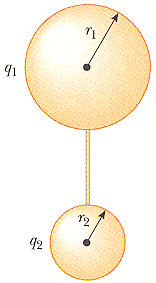# Electric potential question.

## Homework Statement

Two spherical conductors of radii r1 and r2 are separated by a distance much greater than the radius of either sphere. The spheres are connected by a conducting wire as shown in the figure. The charges on the spheres in equilibrium are q1 and q2, respectively, and they are uniformly chargedWhat if initially a charge 2.5 C is put on shell #1 with radius 4.3 m, then a far away shell #2 (initially neutral) with radius 7.3 m is connected to shell #1 by a long conducting wire.
(a) What is the final charge on shell #1?

(b) What is the final charge on shell #2?

(c) What is the electric potential V on shell #1?

(d) What is the electric potential V on shell #2?

V=ke(q/r)

## The Attempt at a Solution

I know that the electric potentials of the two shells are equal due to the conducting wire. It says that the conducting wire is "long" so I believe they behave like point charges and I would use the equation I listed. So I figured I would set the equations for the two shells equal and solve, like so:

ke(q1/r1) =
ke(q2/r2)

and solve for the unknown q. I thought this would give me the answer to part A but I was marked wrong... can anyone tell me what I'm doing incorrectly?

Thanks

Last edited: